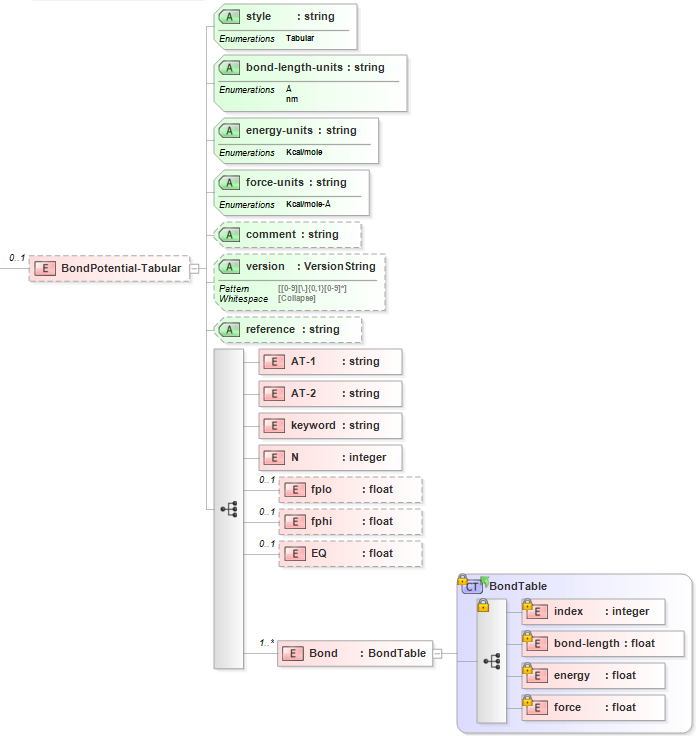3.5. Tabular Bond¶

3.5.1. Tabular Form¶

The tabular bond potential has the parameters:

 Parameter Parameter Definition Units N Number of tabulated values N/A FP Derivatives of the force at the innermost (fplo) and outermost (fphi) bond lengths force/length EQ Equilibrium bond length length

The tabular bond potential has the tabulated values:

 index bond-length energy force i_1 bl_1 e_1 f_1 … … … … i_N bl_N e_n f_N

3.5.2. XML Schema¶

The XML schema for the tabular bond potential has the following representation (design mode representation using Liquid XML Studio):The relationship between the parameters/symbols and XML schema notations are given by:

 Parameter Definition Parameter/Symbol Schema Notation Atom type of atom [i] $$i$$ AT-1 Atom type of atom [j] $$j$$ AT-2 Section identifying keyword N/A keyword Number of tabulated values N N Derivative of the force at the innermost FP fplo Derivative of the force at the outermost FP fphi Equilibrium bond length EQ EQ Index index index Bond length bond-length bond-length Energy energy energy Force force force

The general attributes (describing the entire data set) are given by:

 General Attributes Cardinality Value/Definition style Fixed Tabular bond-length-units Required Enumerations specified in schema energy-units Required Enumerations specified in schema force-units Required Enumerations specified in schema comment Optional Comment attached to parameter set version Optional Version number of parameter set reference Optional Reference attached to parameter set

Note that an XML document will be rejected from being entered into the WebFF database if a required attribute is left unspecified.